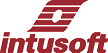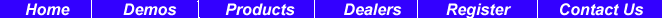Newsletter Issue #66, February 2002

# Rubberbanding Key New Feature in 8.x.10 ICAP/4

Intusoft demonstrated rubberbanding and other significant new features in its latest ICAP/4 Windows 8.x.10 release at DesignCon 2002 in Santa Clara on January 29-30th.

ICAP/4Windows features SpiceNet, an integrated schematic capture program; IsSpice4, the only SPICE simulation engine with true interactive features; extensive model libraries, and IntuScope5 waveform analyzer.

Intusoft’s latest 8.x.10 version of ICAP/4 software offers these new features:

• Rubberbanding — This new feature allows you to move a set of components without breaking associated wiring connections. The ALT key acts as a toggle ON/OFF switch to enable and disable this function.

• One-Click Advanced Parameters Access — Now you can access Standard, Monte, Optimize, Sweep, Parameters, and Tolerance in one easy button click on the main Simulation Control Dialog box.
 In This Issue 1 5 Model Theory 7 Demo Enabled Models 9 Z-Transform Bode Plot 10 Test Designer Key to Structural Integrity 15 New SPICE Transistor Models
• Send a Script to Scope or Spice directly from the Schematic — Use new SendScript feature to send an ICL script from the schematic directly to Scope5. From the schematic, select A to get into text mode, type your script, right click the mouse button and select Send Script to send the script to Scope5. The script will be automatically run in the Scope5 waveform analyzer.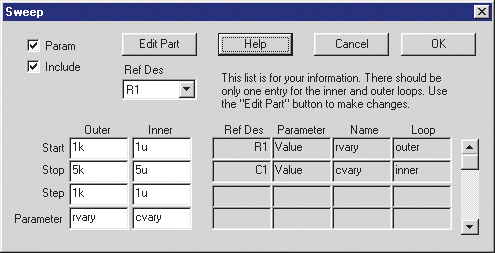Easily Add Sweep and Optimize Part Parameters — Within Sweep and Optimize dialogs you can now select from a part list and edit part parameters without leaving the dialog box.
 Better Optimizer Information — After you enter a percent tolerance for the optimized parameter, you are able to view the minimum, maximum and mean value for that parameter.Modified B Element — Added the capability to copy “B Element” expressions to the part comments, so they will be displayed on the drawing. An Enter dialog was added for ease of entering very long expressions.

New ICL Scripts Added — Expandx, expandy, and expandxAllPlots ICL scripts were added to let you easily modify your waveform viewing area. Family script was added to create eye diagrams. Superfamily script combines multiple vector tags into one to reduce presentation clutter. Drawing scripts allow you to easily format waveform graphs.

Easy Location of Subcircuit Vectors — The Add Waveform Dialog in IntuScope now features an expandable tree structure for subcircuit vectors.
 Dynamic Help Menu For Scope5 — HELP now includes updated Movie Tutorials, and web access to the latest documentation.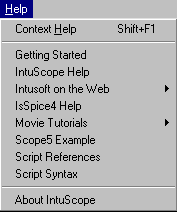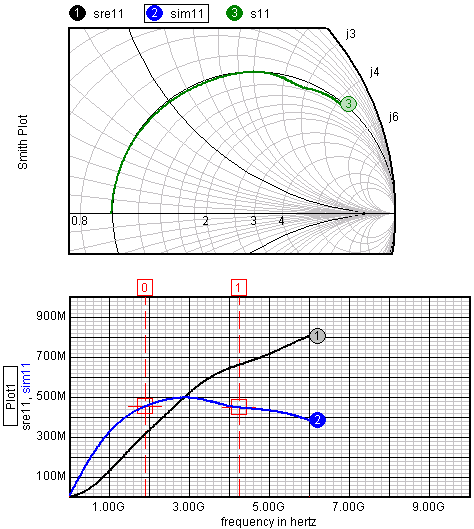Smith Charts — As a Test Designer, ICAP/4 Professional, or RF user, you can now plot Smith Charts by reading in a Touchstone formatted file. Smith Charts may be used to calculate impedance determination, impedance matching, noise figure optimization, or stability.

# Model Theory

The forward converter Z-Transform model extends the approach used by Yaakov  in which behavioral relationships were directly implemented. Rydley  demonstrated the need for modeling transport delay. However, in this case, a Z-Transform model implements this affect directly. Various loss mechanisms can be added to the large signal model in order to predict DC-DC converter efficiency using an operating point analysis. The following model elements will be discussed and the behavioral equations developed.

 Table 1. Efficiency Measurement Rload Pload From OP Analyses From TRAN Analyses .2 125 62.5% 62.7% (Ron set to match) .7 37.5 81.8% 81.8% 1 25.0 84.6% 84.4% 2 12.5 88.1 87.8 4.7 5.3 89.5% 89.5% 10 2.5 88.6% 88.8% 20 1.25 85.6% 87.2%

Model Elements

1. Sampling theory
2. CM/VM Control law
3. Fraction Order Hold
4. Z Transform Integrator
5. CCM/DCM mode switching
6. Input and Output current/voltage equations
7. Magnetizing inductance effects
8. Leakage inductance induced losses
9. Transistor switching loss
10. Switched capacitor loss model
11. Resistive Losses
12. Core Loss

Sampling Theory

To achieve a large signal model, the cycle-by-cycle switching difference equation must be derived. It is not suitable to assume conservation of power through the converter at each instant because the reactive elements store and release energy. Z-Transform theory predicts the time domain performance at each sampling instant. For the class of PWM circuits, it is convenient to set this instant at the beginning of each pulse as shown in Figure 1a and 1b. This is not very satisfying to the end user because it under-estimates the output current. To overcome this we calculate an average output current based on our knowledge of the sampled data state.

Z Transform Integrator

The waveforms for DCM and CCM are shown in Figure 1a and 1b, respectively. We begin each cycle with the previous sample current, I1. The calculated result at the end of the cycle, Io, is fed into the input of the transmission line. The sample delay, Z^-1, is e^sT in continuous time and it can be simply modeled as a transmission line. The transmission line works for both AC and Transient analysis, thereby preserving the small signal characteristics needed for stability analysis and also the large signal description needed for the transient case. Idischarge will change with the inductor conduction mode. The representation in Figure 1c is actually a bootstrap integrator. If the gain exceeds 1.0, the output will grow to infinity. The gain was set to .995 to prevent numerical round off from making this circuit unstable. Reducing Gmin increases numerical precision. As Gmin is reduced, the integrator gain can be made to approach 1.0; however, DC convergence will eventually fail for sufficiently small Gmin.

#Figure 1a. The Z-Transform sampling instant is shown at the beginning of each pulse of this class of PWM circuits. Figure 1a shows the waveform for DCM, where lout = Ddis*IDischarge/2.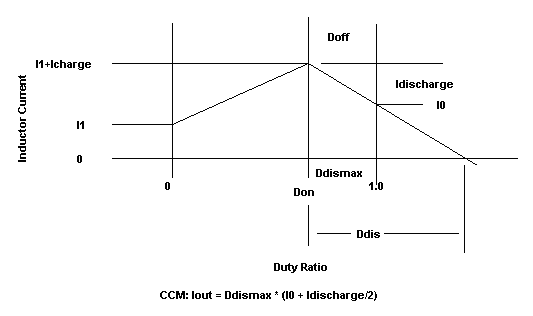Figure 1b. The Z-Transform sampling instant is shown at the beginning of each pulse of this class of PWM circuits. Here the waveform for CCM, where lout = Ddismax * (10 - Idischarge/2), is shown.

CCM/DCM Mode Switching

The terms used in Figure 1a and 1b are defined as:

 Don The fraction of the period the switch is ON. DDIS The number of periods to fully discharge the inductor DDISMAX The time available for discharge in the current period. If Ddismax > Ddis then the mode is CCM, else its DCM. 1-2 MegHz switching Voltage Regulator Module.

 DDIS = 1 – Don Icharge = (Vin-Vout)*Don/(L*F) If (Mode == CCM) Idischarge = (I1 + Icharge)* DDISMAX / DDIS else Idischarge = Vout * DDIS/(L*F)

You’ll find these equations modified slightly in the actual model to account for snubber delay and to prevent divide by zero during numerical iterations. Figure 2 illustrates how that was done. Notice that the ModeCCM switch has a small hysteresis in order to prevent numerical oscillation. You can see that variables like Don are nodes so that the node voltage, used in the equations, becomes V(Don).

#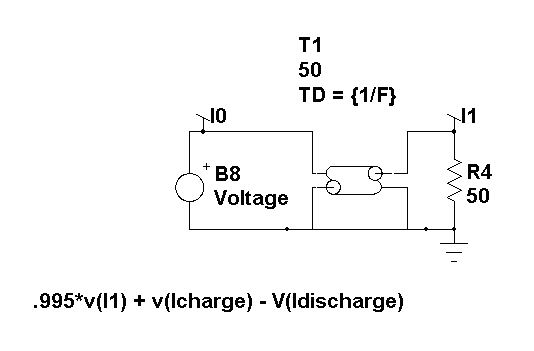Figure 1c. A transmission line models Z^-1.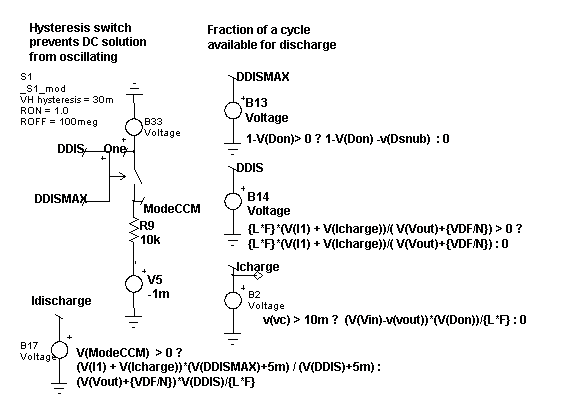Figure 2. Calculating average output current.

Output Current/Voltage Equations

Figure 3 shows the method to calculate average output current. These currents are sent to the output in 2 parts; charging and discharging. The charging component (B24) is dependent on Don while the discharging part (B4) is controlled by the mode. In both cases, the average is taken so the output voltage and currents will be at their average values rather than the sample data points. Diode, D2, prevents negative output. It has a low emission coefficient, 3m, which has its internal temperature set to 27 deg. C. independent of circuit temperature. One of the general problems with this model is that it can find negative solutions that have no interest. It is even possible for a positively clamped state variable to have a negative result computed during the matrix solution. These negative values can result in instability as well as divide by zero overflows. That’s why you see many expressions with positive clamped results (expression > 0 ? expression : 0).

#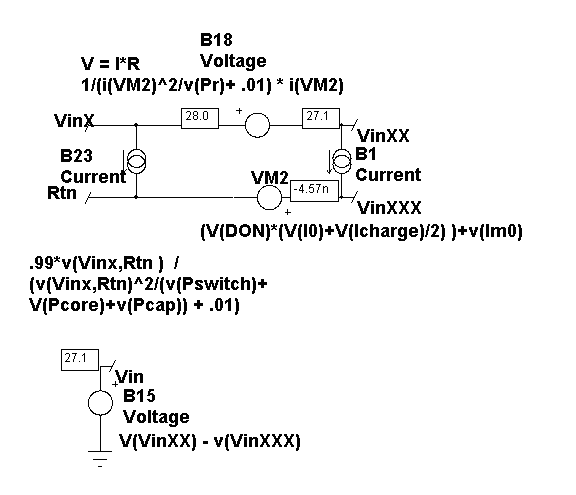Figure 4. The input voltage and current equations.

On the input side, illustrated by Figure 4, conduction occurs only during Don. B1 computes the basic relationship. Notice the magnetizing current, Imo, term. Its equations will be developed later on. B23 and B18 introduce losses. B18 reduces the input voltage, simulating resistive losses in the power switch and transformer. B23 accounts for leakage inductor, core and switched capacitor losses. B18 and B23 are used to model resistive and conductive losses using models that are well behaved around zero. The following equation for B18 shows how the parameter del prevents a singularity at zero current while squaring the current in the denominator forces a the term to be positive, thereby eliminating any chance for a divide by zero. Vin is referenced to simulator ground, allowing the external inputs to be offset from ground.

 V = I*R = I(VM2)*1/(1/R + del), I^2R=P; R = P/I^2 V = I(VM2)*1/(I(VM2)^2/P + del)

CM/VM Control Law

The control signal, Vc, is converted from an external ground on the input side using B10. The control signal is processed through a fractional order hold, accounting for transport delay. This FOH and the Z-Transform integrator realistically model the phase degradation near one half the sampling (Nyquist) frequency.

The control equations are implemented as shown in Figure 5.

RB is the current sense resistor and the equations assume that 1 volt will cause the maximum current flow. Setting the parameters RB=0 and MC=1 will result in voltage mode control where the duty cycle is set by v(Cntrl).

 For CM controllers, the following equations apply: I1+Icharge+Imag = Vcontrol/Rb Icharge = Don*(Vin-Vout)/(LF) I1 + Don*Vin/(L*F) + Imag = Vcontrol/Rb Don = (Vcontrol/Rb - I1-Imag)*(L*F)/Vin Don = (Vcontrol - Rb(*I1-Imag))/(RB*Vin + MC) To operate in VM set: MC=1, RB=0 For CM MC = slope compensation as fraction of the slope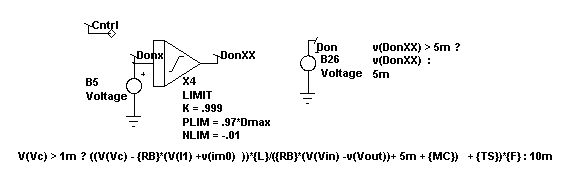Figure 5. Control law for both CM and VM controllers.

# Demo Enabled Models

We have many requests from semiconductor vendors to increase the size limit on our demo software so that they can show how new ICs work. The IC models and circuits have become so complex that to increase our complexity test so these circuit simulations would run amounts to giving our software away. In order to accommodate the showcasing of these products, Intusoft invented a method to exempt certain portions of a drawing from the IsSpice complexity test. This allows a simple circuit configuration to simulate but prevents it from working when the user adds parts and functionality needed to make a production circuit.

Here’s how it works. Right after the .subckt line in the Spice netlist, this line is inserted in the netlist:

*exempt arg1 arg2 arg3

This causes the demo version of IsSpice to scan the remainder of the subcircuit netlist. If the subcircuit netlist passes the scanning test, then the subcircuit is not processed by the complexity testing procedure. The scanning method prevents topology changes in the subcircuit from being accepted by the simulator. Each subcircuit is scanned according to the rules presented in its *exempt line so that *exempt lines can’t be applied to other subcircuits.

We do this to showcase our software so that the combination of software and models is synergistic. The whole is more valuable than the sum of its parts, or so we hope. You will see this feature in SpiceNet drawings from ON Semiconductor and TI in the next few months.

# Z-Transform Bode Plot

Intusoft recently did a Bode plot for an internal loop model using our new Z-Transform model for the switched inductor.

The Z-Transform model shows gain greater than 1 at harmonics of the switching frequency. This behavior suggests instability that would fold back below the power supply Nyquist frequency.

Note: The frequencies above the Nyquist frequency are generally taken to mean that these frequencies fold back beginning at ½ the switching frequency, extending into negative frequencies until reversing. This behavior is well known and used in heterodyne detection systems.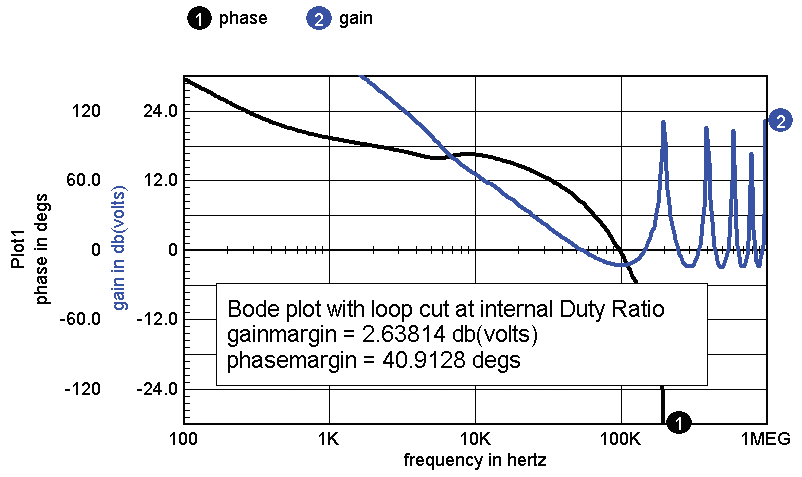Figure 6. Bode Plot with Loop Cut at Internal Duty Ratio.

There is no evidence of instability in the outer voltage control loop, as shown in Figure 7.

The switching models transient output voltage shows ripple primarily at the switching frequency; however, there is a small chaotic noise component. This is shown in Figure 8.Figure 7. Bode Plot with Loop Cut at Voltage Control.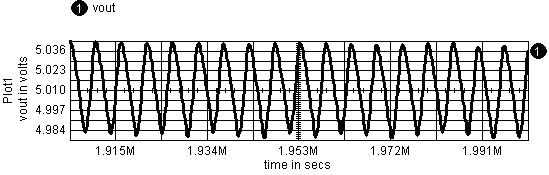Figure 8. Switch model's transient output voltage shows ripple at the switching frequency.

Questions for Future Study

1. Is the unexpected transient ripple really there or is this just numerical noise?
2. What is to be made of the AC response above the switching frequency?

# Test Designer Key to Structural Integrity

Test Designer is software which designs test strategies to assure structural integrity. There have long been arguments over structural versus functional testing; however, structural test MUST be performed to assure reliability. Here’s why:

Did You Know? You've designed redundant or fault tolerant systems in order to ride through a single point failure. But over time it is increasingly likely that a failure has occurred and that if undetected a catastrophic event is likely. The availability of redundant systems is fundamental to avoiding catastrophe. Either built in self test, BIST or auxiliary testing is needed to perform structural testing that assures proper component operation. This kind of testing effectively resets the accumulated failure rate to zero for each part that passes the test. After a successful test; then when you begin a mission, you have full advantage of the redundant systems.

How is it Done? It is necessary to analyze the results of each likely component failure. Then a test strategy is necessary to detect each fault. Work done in the USA for commercial and military aircraft systems has produced methods for sequencing these tests to produce a decision strategy that isolates faults. Putting this together with simulation technology, it is possible to complete this apparently monumental task.

Say that Again Undetected part failures make a catastrophic event more and more likely the longer a system operates without fault diagnosis. Fault diagnostic test design is possible today using simulation technology and established test design techniques. That combination has been put together with Intusoft’s Test Designer. You can even model electro-mechanical components and perform system level diagnostic testing.

Show me an Example Let’s try an automotive steering system. In the near future electrical systems will replace the mechanical-hydraulic method currently in use. The steering wheel may become something like a force feedback joystick. It sends a voltage to a controller and road feedback is transmitted back to the driver. The controller requires input position, output position and a control law. If analog, many resistors and capacitors are used to obtain a control transfer function. If digital, properly functioning A/D and D/A converters and other IC chips must be working. Both require a switch mode power controller to drive an electric motor that steers the car. Using affordable parts, a failure rate of 1 to 100 failures per 100 million hours per part can be expected. That produces about 10 failures per million hours for a system. While that sounds pretty good; if you take 10 million cars for twenty years, you get 17.5 million failures! Even lower production rate systems like commercial and military aircraft are exposed to incredible risk levels. Fault tolerance is no longer an option — it is a requirement. Now here’s the rub; if you want a mission success of 99.99% (that’s not unusual for taking a trip to the store and returning alive!), then you can’t go for long without testing the redundant systems in order to purge broken parts. It’s no longer just an oil change every 3000 miles!

# New Spice Transistor Models

 DarNPN(8) 2N6283, 2N6284, 2N6301, 2N6350, 2N6351, 2N6353, 2N6384, 2N6385 DarPNP(3) 2N6649, 2N6650, 2N6650 NPN(27) 2N2060, 2N2060L, 2N2219AL, 2N2222AU, 2N2432, 2N2919, 2N2919L, 2N2920L, 2N3019S, 2N3418, 2N3418S, 2N3419, 2N3419S, 2N3420, 2N3420S, 2N3421, 2N3421S, 2N3501L, 2N3735, 2N3735L, 2N4150, 2N4150S, 2N5237S, 2N5238S, 2N5662, 2N5663, 3N3439 PNP(24) 2N2605, 2N3251A, 2N3468, 2N3468L, 2N3634L, 2N3635, 2N3635L, 2N3636L, 2N3637, 2N3637L, 2N3763, 2N3763L, 2N3764, 2N3765, 2N3810, 2N3810L, 2N3811, 2N3811L, 2N3867, 2N3867S, 2N3868, 2N3868S, 2N5416, 2N5416S PowNPN(36) 2N2151, 2N2814, 2N2880, 2N3442, 2N3716, 2N3739, 2N3749, 2N3996, 2N3997, 2N5004, 2N5038, 2N5039, 2N5157, 2N5237, 2N5238, 2N5250, 2N5251, 2N5302, 2N5660, 2N5664, 2N5665, 2N5666, 2N5667, 2N5672, 2N5685, 2N5686, 2N6033, 2N6249, 2N6250, 2N6251, 2N6308, 2N6338, 2N6546, 2N6547, 2N6676, 2N6678 PowPNP(11) 2N3740, 2N3741, 2N4399, 2N5005, 2N5683, 2N5684, 2N5745, 2N6211, 2N6212, 2N6213, 2N6438

 Ben-Yaakov, S. “Average Simulation of PWM Converters by Direct Implementation of Behavioral Relationships,” IEEE Applied Power Electronics Conference (APEC, 1993), pp. 510-516

 Ridley, R. B. “A New Small-Signal Model for Current-Mode Control,” PhD. dissertation, Virginia Polytechnic Institute and State University, 1990.

BACK TO THE TOP